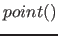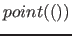Next: Properties Up: Fib elements Previous: Vectors   Contents   Index

### Point

For the description of the point element see section 9.2 on page.

A normal point element has the name `point`. A point without a position vector () has also the name `point` but contains no position vector. If the point is for the entire background (: point in which the position vector has no elements), the name of the XML element is `background`.

Normal points included a position vector (see section 22.3.1). The number of vector elements is determined by the number of dimensions (see section 22.2.3 on page) the number of possible values for each position vector element, is determined by the domain of the dimension (see section 22.2.3 on page).

An example for a normal point:

```<point>
<vector type="position">
<variable>2</variable>
<value>21</value>
</vector>
</point>
```

An example for a point with no impact respectively a point with no position vector:

```<point/>
```

An example for a point with an empty position vector for the entire background:

```<background/>
```

Betti Österholz 2013-02-13